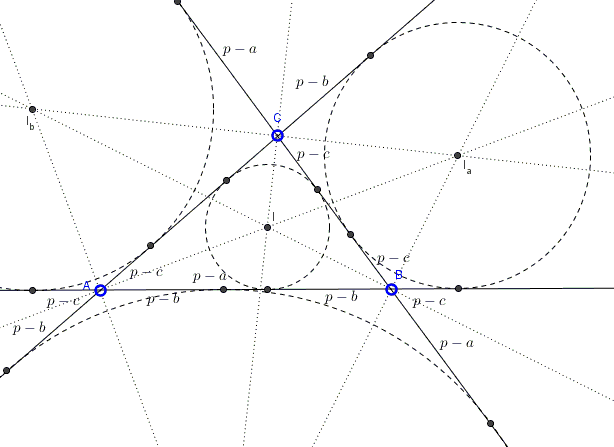# Conics Related To In- and Excircles

### Problem

The points of tangency of the excircles on the extensions of the sides of a triangle lie on a conic. So do the points of tangency of the incircle and excircles on the sides proper:### Hint

These are rather obvious applications for Carnot's Theorem.

### Solution

For a proof I shall simply refer to the diagram below, with the segment lengths derived on a separate page:Carnot's criterion

$\displaystyle \bigg(\frac{AC_a}{BC_a}\cdot\frac{AC_b}{BC_b}\bigg)\cdot\bigg(\frac{BA_b}{CA_b}\cdot\frac{BA_c}{CA_c}\bigg)\cdot\bigg(\frac{CB_c}{AB_c}\cdot\frac{CB_a}{AB_a}\bigg)=1.$

for the six tangencies of the excircles on the side extensions appears as

$\displaystyle \bigg(\frac{p-c}{p}\cdot\frac{p}{p-c}\bigg)\cdot\bigg(\frac{p-a}{p}\cdot\frac{p}{p-a}\bigg)\cdot\bigg(\frac{p-b}{p}\cdot\frac{p}{p-a}\bigg)=1,$

while the six tangencies of the incircle and the excircles on the sides proper it appears as

$\displaystyle \bigg(\frac{p-b}{p-a}\cdot\frac{p-a}{p-b}\bigg)\cdot\bigg(\frac{p-c}{p-b}\cdot\frac{p-b}{p-c}\bigg)\cdot\bigg(\frac{p-a}{p-c}\cdot\frac{p-c}{p-a}\bigg)=1,$

where, as usual, $p=(a+b+c)/2.$

### Acknowledgment

There is a very comprehensive page at the site of Ricardo Barroso Campos devoted to the work of Lazare Carnot and, his criterion, in particular.